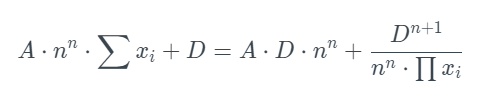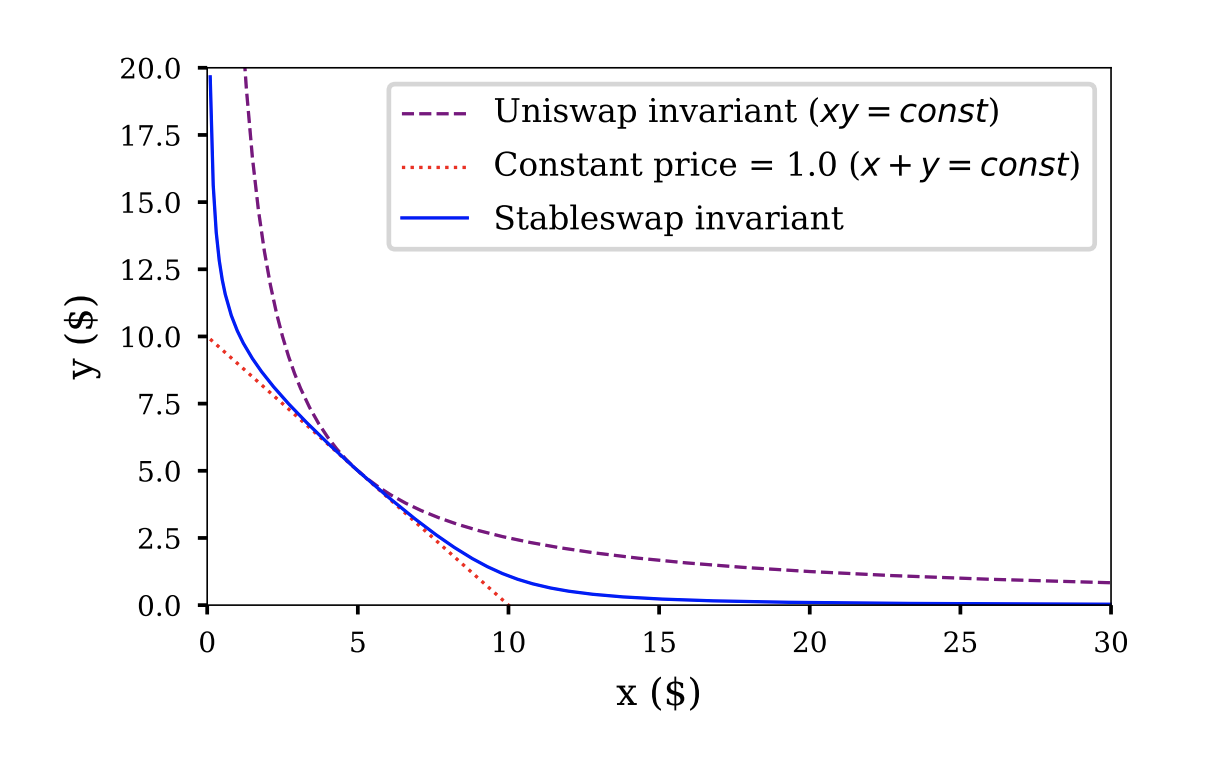# Stable Pools

## Overview

Stable Pools are designed specifically for assets that are expected to consistently trade at near parity, such as different varieties of stablecoins or synthetics. Stable Pools use Stable Math (based on StableSwap, popularized by Curve) which allows for significantly larger trades before encountering substantial price impact, vastly increasing capital efficiency for like-kind swaps.
Please note that unlike with weighted math, the assets in the stable pools must have the same weights. For example, if a stable pool contains 5 assets, any asset in the pool must have a 20% share.

### Low Price Impact

Traders enjoy tighter spreads and lower price impact. These pools allow for larger trades of assets before encountering a significant price impact.

### Stable Swaps Under the Polaris Hood

One of the key advantages to having Stable Pools on Polaris specifically is that they are plugged into the same protocol as all other pools. Swapping between stable coins is frequently used for arbitrage when one token is paired with two different stable coins in different pools. By leveraging Batch Swaps on Polaris, these trades can be combined into a single, gas efficient transaction.

## Maths

Stable Math is designed to allow for swaps between any assets that have the same price, or are "pegged" to the same asset. The most common examples are stablecoins that track US Dollars (USDT, USDC), and assets that track the price of Bitcoin (wBTC, renBTC, sBTC). Prices are determined by the pool balance, the amplification parameters, and amounts of the tokens that are being swapped.
In an ideal scenario, it would make sense to simply allow 1-to-1 trades for these assets; this would be a constant sum curve. In a worst case scenario where one or more of these assets loses their peg and their value diverges, it would make sense to enforce trade rules for uncorrelated assets; this would be a constant product curve, such as the one outlined in weighted math.
Since most real-life cases do not fall in either the ideal or disaster category, the stable math curve combines the constant sum and constant product curves and is designed to facilitate approximately 1-to-1 trades that incur large price changes only when token balances differ greatly. The amplification parameter, A, defines the degree to which the stable math curve approximates the constant product curve (when A=0), or the constant sum curve (when A→∞）
Constant sum or linear invariant: ∑xi=const
Constant product invariant: ∏xi=const
where xi represents the balance of the token in the pool.
Stableswap invariant is a combination of the above two and offers a much lower price slippage than Uniswap for stablecoin pools. It is given by:A is the amplification coefficient and D is the invariant. Please note that unlike with weighted math, the assets in the stable pools must have the same weights. For example, if a stable pool contains 5 assets, any asset in the pool must have a 20% share.The figure above is a simplified version of the stable pool, containing two assets. The stableswap invariant is a combination of the constant sum and constant product invariant. Check here to see the impact of different parameters on the stableswap invariant curve.
The lower the amplification coefficient is, the closer to the constant product model the curve will be, as in Uniswap. Normally, trades will have relatively more significant slippages.
The higher the amplification coefficient is, the closer to the straight line the curve is, which is the case in the linear invariant. Normally, trades will have lower slippage. But when one token’s market price deviates from the peg, it will lead to a massive change in the pool’s composition.